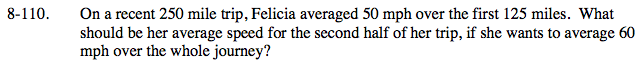### Home > PC > Chapter 8 > Lesson 8.2.3 > Problem8-110

8-110.$\text{Average Rate}=\frac{\text{total distance}}{\text{total time}}$

$\text{Average Rate}=\frac{250}{t_{\text{first}(125)}+t_{\text{second}(125)}}$

$\text{Average Rate}=\frac{250}{\frac{125}{50}+\frac{125}{r_2}}$

$60=\frac{250}{\frac{125}{50}+\frac{125}{r_2}}$

Multiply both sides by the denominator:

$\left(\frac{125}{50}+\frac{125}{r_2}\right)(60)=250$

$\frac{(125)(60)}{50}+\frac{(125)(60)}{r_2}=250$

Multiply each term by 50r2.

$(125)(60)(r_2)+(125)(60)(50)=250(50\textit{r}_2)$

375000 = 5000r2

r2 = 75 mph